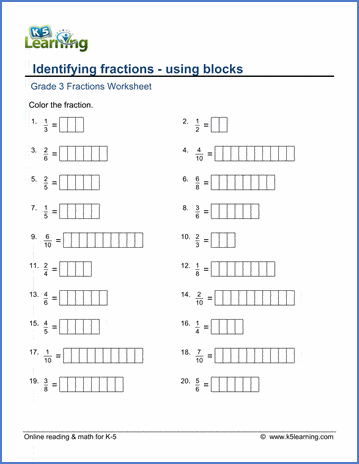# Identifying fractions

## Worksheets: Fractions & decimals - identifying fractions using blocks

Below are six versions of our grade 3 math worksheet on identifying fractions using blocks.  Students are given a simple fraction and asked to color in blocks to make a pictorial representation of it.  Students learn that the denominator (bottom number of the fraction) represents the number of blocks, and the numerator represents the number of blocks to be colored in.  These worksheets are pdf files.## More fractions worksheets

Explore all of our fractions worksheets, from dividing shapes into "equal parts" to multiplying and dividing improper fractions and mixed numbers.

## What is K5?

K5 Learning offers reading and math worksheets, workbooks and an online reading and math program for kids in kindergarten to grade 5.  We help your children build good study habits and excel in school.